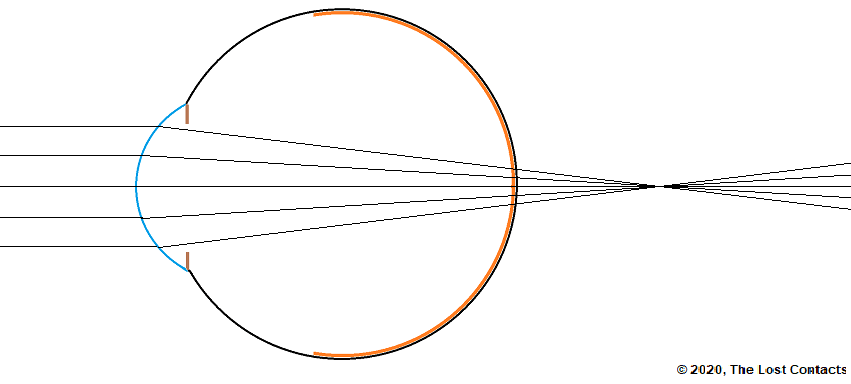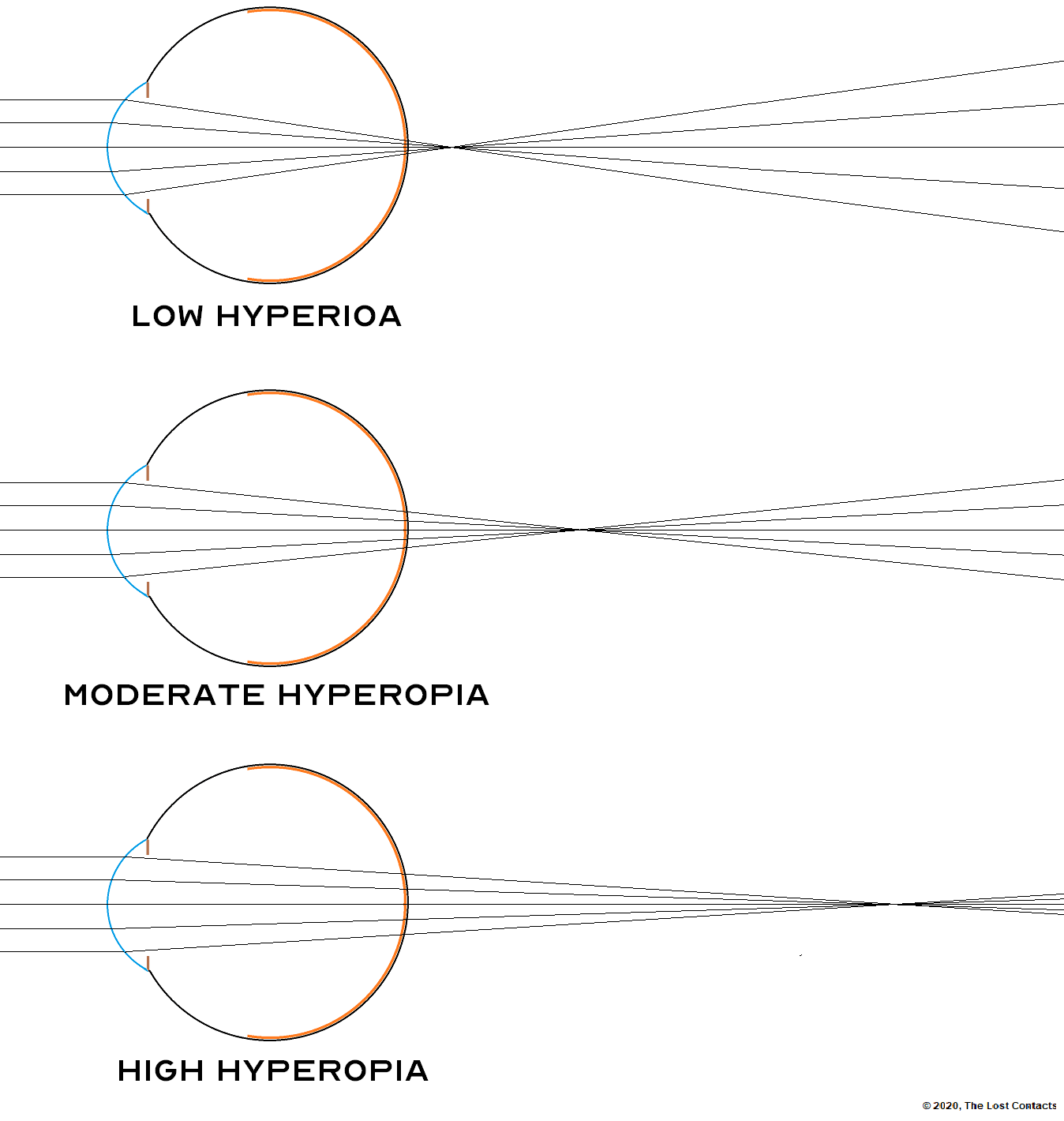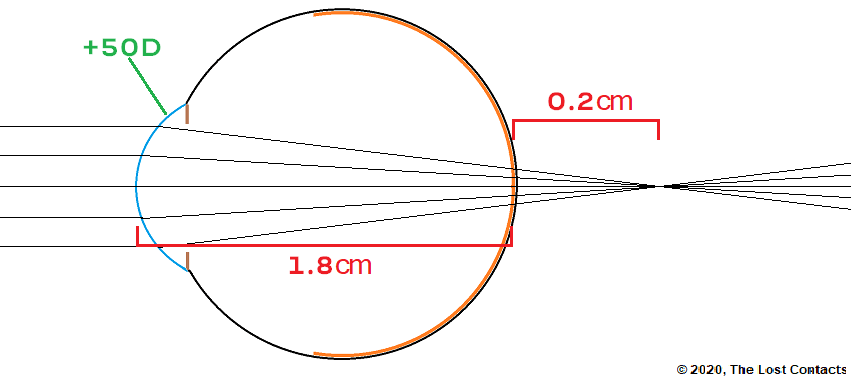This is lesson 7 in this course. Please review lessons 1-6 if you haven’t already done so.

# Hyperopia

### What is Hyperopia?

Hyperopia, or hypermetropia, is the refractive condition that results when the focal point of parallel light entering the eye forms behind the retina.

The word hyperopia is the name of the condition and the word hyperopia is used to describe somebody with hyperopia. I.e., She has hyperopia, she is hyperopic.

A more common word for hyperopia is farsightedness. The word farsightedness is the name of the condition and farsighted is used to describe someone who has farsightedness. I.e., He has farsightedness, he is farsighted.

Here’s what hyperopia looks like in a ray diagram:The above diagram decpicts a cross section of an eye with the focal point forming behind the retina. This is just a helpful way of visualizing hyperopia, but in reality, the focal point never actually has a change to form. The light rays are acutally stopped an absored as soon as they hit the retina.

The point is that the light rays are not in focus when the hit the retina, and should they have been able to keep going, the focal point would eventually have formed behind the retina.

Just like in myopia, hyperopia exists in varying degrees of severity. It can be very low or very strong as you can see here:The reason for the focal point forming behind the retina in hyperopia is that the cornea does not converge the light entering the eye enough.

Recall from Lesson 3 that lens power and the focal distance are linked in the following way:

The higher the lens power, the shorter the focal distance.

The lower the lens power, the longer the focal distance.

In the case of hyperopia, the focal distance is too long. To shorten the focal distance, we have increase the lens power.

How do we increase the lens power of the cornea? Well we cann change the shape of the cornea itself, so we have to add lenses in front of it.

Recall from Lesson 5 that the cornea acts as a plus (converging) lens. Theefore, in order to increase the overall power of the eye, we will have to add more plus (convering) lenses in front.

*Note that to correct hyperopia, we are doing the exact opposite as what we do to correct myopia.

To illustrate how adding plus (converging) lenses in front of an eye helps correct hyperopia, let’s consider the following example.In this example, we see parallel light coming from optical infinity, we see that the cornea has a power of +0.50D, the length of the eye is 1.8cm (0.018m) and the focal point is 0.2cm (0.002m) behind the retina.

The question is; what lens power needs to be placed in front of the eye in order for the focal point to land exactly on the retina.Let’s start by establishing the goal. The goal is to have the focal point on the retina. The retina is 1.8cm behind to the cornea. So what total lens power do we need for that?

The formula for lens power is:

lens power = 1 / focal distance

In this example we want the focal distance to be 1.8cm (because that’s how far the retina is from the cornea).

lens power = 1 / 0.018

lens power = +55.5D

This means that for the focal point to be on the retina, the cornea must have a lens power of +55.5D. But it only has a lens power of +50D, not +55.5D, so what do we do?

Well, since the cornea is missing +5.5D of converging power, we will add an plus (converging) lens in front of the cornea with a power of +5.5D!

### Calculations

Total Power Needed = Power of the Cornea + Power of Glasses Needed

+55.5D = +50D + Power of Glasses Needed

Power of Glasses Needed = +55.5D – (+50D)

Power of Glasses Needed = +5.5DSo in this example, with a +5.5D plus lens in front of this eye, the light is perfectly focused on retina!

Just like in our example from Lesson 6, this is not exactly how optometrists figure out what lenses will make the vision better. They don’t know what the length of the eye is so these calculations are not possible in the exam room. Instead, they just test different powered lenses in front of the patient’s eyes until they find the one which gives the best vision (… in a nutshell).

## Pop Quiz!

Ok, now that we have a better undesrstanding of hyperopia, try to answer this question based on everything you know so far.

Why do farsighted people (people with hyperopia) have more difficulty seeing up close than in the distance?

Hint: Think of how light behaves when it comes from a distance vs when it comes from up close.

Leave comment below to find out the answer!

We have now learned a lot of theory in this course. If you have any questions about any of the material so far it’s very important that you clear it up before the next lesson. You can leave your questions in the comments section of any of the lessons.

The reason I bring this up is because the next lesson will really test your understand of the concepts we’ve learned so far. It’s one of the most difficult refractive conditions to grasp and the vast majority of people have no idea what it is. I’m talking about astigmatism. So get prepared, and then move onto Lesson 8!

==>The Optics of Vision – Lesson 8: ASTIGMATISM <==